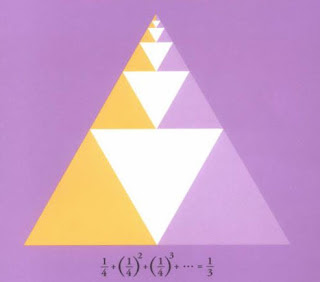## Friday, 25 July 2008

### Proof Without WordsI have stated previously how much I like "napkin" techniques that give quick calculations or estimates of a problem. I also like things like visual displays which essentially prove some mathematical idea. The one at the top of the page is from the cover of Roger Nelsen's "Proofs without words II.." which is way too expensive for a paperback, but I will probably break down and buy it.

The problem, is that, except for mathemtaiticans who already know the proof, it seldom convinces "without words". Most of my high school students will not look at this image and be able to explain easily and clearly why it shows that the limit as n goes to infinity of 1/4 + (1/4)2 + (1/4)3+ ... +(1/4)n is equal to 1/3. If I'm wrong, not generally, but in your particular case, then stay with me and read what's written, and tell me if you see it the way I do.

I think they will be able to see that the triangle is divided into thirds... by the colors, 1/3 purple, 1/3 orange, 1/3 white. But I don't know if they can see the series of powers of 1/4 going off to infinity. That's why they have high school teachers... and so here are some words to help make it more "visual"

Look at the largest white triangle... can you see it is 1/4 of the largest (outside) triangle?... Ok, now look at the line across the top of the biggest white triangle, it connects the midpoints of two legs of an equilateral triangle, so the triangle above this medial segment, the one with multiple smaller triangles in it, is exactly congruent to the Biggest white triangle and is 1/4 of the total area of the outside triangle also. This upper triangle is a scale model of the original outside triangle,with all the same colors in the same positions and the white triangle in it is 1/4 of the area of this upper replica. So the second largest white triangle is 1/4 of the area of the Largest white triangle.... its area is 1/16 or (1/4) 2. Now the line above the second smallest white triangle is a medial segment of the upper triangle, and so the triangle above it, which is also a scale model of the original biggest triangle, is also 1/16 of the total area... and the third smallest white triangle, is 1/4 of that, so its area is 1/4 of 1/16 or (1/4)3... OK, now you see it, and as you move out each white triangle is 1/4 of the previous one... and sure enough, all the white triangles add up to 1/3 of the total area.

If you are one of my students, next year remind me to say "similar triangles" a lot...if you are going to be in calculus, don't worry, we will